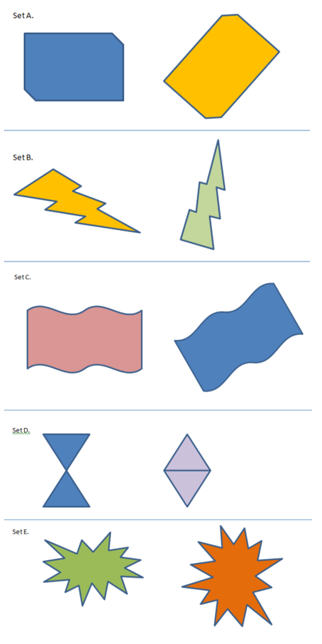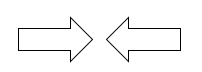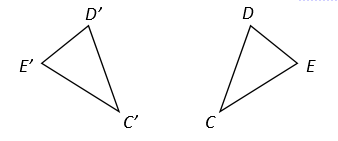# Transformations and Angle Relationships

## Objective

Understand the rigid transformations that move figures in the plane (translation, reflection, rotation).

## Common Core Standards

### Core Standards

?

• 8.G.A.1.A — Lines are taken to lines, and line segments to line segments of the same length.

• 8.G.A.1.B — Angles are taken to angles of the same measure.

• 8.G.A.1.C — Parallel lines are taken to parallel lines.

• 8.G.A.2 — Understand that a two-dimensional figure is congruent to another if the second can be obtained from the first by a sequence of rotations, reflections, and translations; given two congruent figures, describe a sequence that exhibits the congruence between them.

## Criteria for Success

?

1. Understand different ways that figures can be moved while maintaining their original shape and size through translation, reflection, and rotation.
2. Define and prove congruence between two figures; if a figure can be moved to overlap perfectly with the other figure, without stretching or breaking it, then those two figures are congruent.

## Tips for Teachers

?

• This lesson introduces students to the concept of rigid transformations. Students will have prior-learned language to describe these movements, like “flip,” “turn,” or “slide.” Allow students to use these descriptions to enable them to talk freely about what they see; however, be sure to introduce the formal names of these movements so students can begin to internalize them.
• The following tools/materials are needed for this lesson: patty paper (or transparency paper), rulers, scissors, and a teacher computer for Anchor Problem #1. Students may also request access to tape, graph paper, and protractors (MP.5).

#### Fishtank Plus

• Problem Set
• Student Handout Editor
• Vocabulary Package

## Anchor Problems

?

### Problem 1

Show the first video of Ms. Pac-Man under “The Situation.” from Robert Kaplinsky's How Did They Make Ms. Pac-Man?

• Ask and discuss: How can you describe Ms. Pac-Man’s movements?

After discussion, introduce the term “translation” and show the next video (“Translations Only”).

• Ask and discuss: What do you think “translation” means? What other movements did Ms. Pac-Man make?

After discussion, introduce the term “reflection” and show the next video (“Translations and Reflections Only”).

• Ask and discuss: What do you think “reflection” means? Is Ms. Pac-Man making any other movements?

After discussion, introduce the term “rotation” and show the next video (“Translation, Reflections, and Rotations”).

• Ask and discuss: How can we get more precise to describe how far she translates, in what direction she rotates, etc.?

Lastly, show the video with the coordinate plane (“Translations, Reflections, Rotations, and Coordinate Plane”).

• Ask and discuss: How does the coordinate plane help us communicate about movements more precisely?

Note, do not have students complete the list of transformations referenced in the link.

### Problem 2

For each pair of figures, decide whether these figures are the same size and same shape. Be prepared to justify your reasoning. You may use mathematical tools to make your decision.#### References

Illustrative Mathematics Same Size, Same Shape?

Same Size, Same Shape?, accessed on Oct. 13, 2017, 1:11 p.m., is licensed by Illustrative Mathematics under either the CC BY 4.0 or CC BY-NC-SA 4.0. For further information, contact Illustrative Mathematics.

Modified by The Match Foundation, Inc.

### Problem 3

How would you move one figure to get to the other figure? What transformations would you use? Are the figures congruent?

Example 1:Example 2:Example 3: Lines $P$ and $Q$ are parallel and are transformed to map onto lines $P'$ and $Q'$.## Problem Set

?The following resources include problems and activities aligned to the objective of the lesson that can be used to create your own problem set.

• Examples where students are given two figures to 1) determine if the figures are congruent and 2) explain using rigid transformations; be sure to include congruent and non-congruent examples, similar to Anchor Problem #3
• Example where either a reflection or a rotation may map one figure to another (see below)
• Malik says that the figure below was reflected, but Shayla says it was rotated. Who is right? Explain your answer.?

Triangle ${{{CDE}}}$ underwent a transformation that created triangle ${{{C'D'E'}}}$.a.    Describe how triangle ${{{CDE}}}$ was transformed to become triangle ${{{C'D'E'}}}$.

b.     What features stayed the same?

c.     What features changed?

d.     Is triangle ${{{CDE}}}$ congruent to triangle ${{{C'D'E'}}}$? Explain how you know.

?